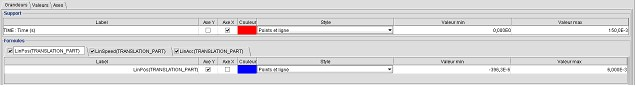# 2D Curve: representation of curves (quantities in X, in Y)

## By default representation

By default, all the calculated elementary curves are represented in the tracing zone.

For each of the curves, the representation is the following:

• on abscissa : the calculation support (path, I/O parameter)
• on ordinate : the calculated quantity (formula with local quantity, formula with I/O parameter) *

The calculated quantities are accessible in the quantity tree (formulas zone).Attention: * If the calculated quantity is a vector local quantity (B, H, ...), the software calculates (and represents) 4 elementary curves (the module, and the three components).

To add/delete an elementary curve in the display zone

• in the Table tab: tick the box « visible » preceding the desired quantity

## Modifying the quantities in X, in Y

To modify the representation of the quantities in X and in Y

• in the tab Table : tick the box Axe X / Axe Y preceding the desired quantity

## Modifying the scales

To modify the extremes of the scales:

• in the tab Axes: choose the min. values, max. values preceding the desired quantity

To pass to the logarithmic scale:

• in the tab Axes : tick the box Log preceding the desired quantity

## Modifying the Style

The user can equally modify the style of the curve displayed by means of the following options:

• by modifying the color
• by modifying the style (line, point, stick, surface…)